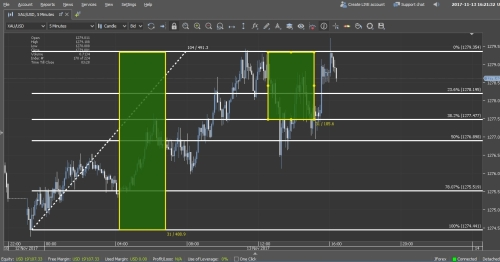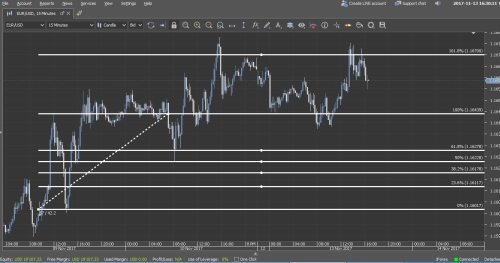The sequence of the Fibonacci numbers is the Italian mathematician Leonardo Pisano. This sequence has been known for centuries. The order is 1, 1, 2, 3, 5, 8, 13, 21, 34, 55, 89 forever. The numbers are calculated by the sum of the previous two numbers.
Example: 1 + 1 = 2 or 3 + 5 = 8
This numerical relationship has been used in financial markets for more than a century. The most important gold number is Phi. Found with gold ratio. This ratio is the ratio of the number of two consecutive numbers. High number share, low number is share. The result is always approximately 0.618, as seen below.a = 0.618
b = 0.382
0.618 / 0.382 = 1.618
Gold ratio: 0.618 + 0.382 = 1.000

There are many Fibonacci rates and methods used for your account, but we will lean on those that have practical functions for your financing transactions. They are used to find potential resistance and support levels of the price of an asset on the basis of Fibonacci retracement. F is based on the principle that the yacht will be drawn in a predictable position and then proceed in the original direction. Historically, the most commonly used remedies were in the areas of 38.2%, 50% and 61.8%. These levels appear to be the point of return for the market and the focus of the majority of analysts.
As we have seen in this gold chart, the price is advancing to the range of \$ 1,190. In the end, it falls to 38.2% and rises again.On the upside, you see a period of 15 minutes for GBP / USD. As can be understood, the market started to retreat at 38.2%, but it has not progressed to a decline of 50%. Prices continue to rise here, reaching new heights.You can always find similar technical analysis tools such as Fibonacci retracement on our dukascopy platform. You have to select the item you want to process by opening the chart window for this. Then press the "Fn" button on the left side of the graphic and click on the first button on the right.
The measurement should be in the same direction as the price movement. Later horizontal lines can be added to the graph. These lines are potential support and resistance levels.
Preferably, you can run the most extreme points of market trends using candlestick charts. Often the difference is insignificant but sometimes crucial. In the following examples you will see that the Fibonacci correction is added on the candle shadows, the trendin peak and the bottom.Fibonacci retracements can be very useful for trend entry. This trend is part of the investment strategy. In this strategy investors observe some important price levels. They open low-risk transactions in the trend direction. In this scenario, you predict prices that will shift in trends from Fibonacci levels. For example, before the price recovered, it declined to 61.8%.The same can be seen on the next chart as a long period of time before the price declines to 61.8%. This is a sign of last resistance levels and a trend towards the market trend.
Translate to English Show original